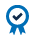## Options Delta

Futures contracts can be an effective and efficient risk management or trading tool. Their performance is basically two-dimensional, either you are up money or down depending on the entry price point and whether the market is up or down versus your position.

But with options on futures there are more dimensions, or forces, acting on the price or premium of the option.

There are metrics to measure each of these different impacts on the premium of an options.  These metrics are often referred to by their Greek letter symbols and collectively as “the greeks.”

Let’s look at one of the most commonly used Greeks – Delta.

Delta is the change in the option’s price or premium due to the change in the Underlying futures price.

It is some portion of the movement of the underlying. Delta is a percentage measure.

Assume, we have a call option priced at 1.00 and it has a .50 delta. This means whatever the change of the underlying future is, the option will move by 50% of that change. Our underlying futures product moved from 96 to 97.5.  This is a 1.5 point move.   So, our option’s premium will now change by 50% of 1.5 or .75.  Making the option’s new price 1.75

Calls always have positive delta between 0 and 1.00, while puts always have negative delta between 0 and -1.00.

The delta of a futures contract is 1.00.

Traders usually refer to the delta without the decimal point. So, a .40 delta is commonly referred to as a 40 delta.

Being Long a call will result in positive Delta; being short a call results in negative Delta. Conversely, being Long a put results in negative Delta; being short a put results in positive Delta. The absolute value of the Delta also tells the approximate probability that the option will finish in-the-money.

For example, if the option has a delta of 20 it suggests it has a 20% chance of finishing in-the-money.  A delta of 50 suggests it has a 50-50 chance of finishing in-the-money.

If an options delta is less than 50 it is said to be out of the-money.  If the delta is greater than 50 the option is said to be in-the-money.  If the delta is equal or close to 50 the option is said to be at-the-money.

The delta is used in calculating hedge ratios to establish a neutral or delta hedged position using the underlying futures. Let’s say we sold 8 call options that have a 25 delta, we have a delta position of -200. To be delta neutral, we need to buy 2 underlying Futures contract.

Delta is dynamic and changes with movement in the underlying.  That means delta neutral ratios and other hedge ratios using options are also dynamic and change too.

#### ACCREDITED COURSEDid you know that CME Institute classes can fulfill CFA and GARP continuing education requirements? Every CME Institute course can be self-reported in your CFA online CE tracker and select classes can be used for GARP credits. See which of our classes qualify for GARP credits here.